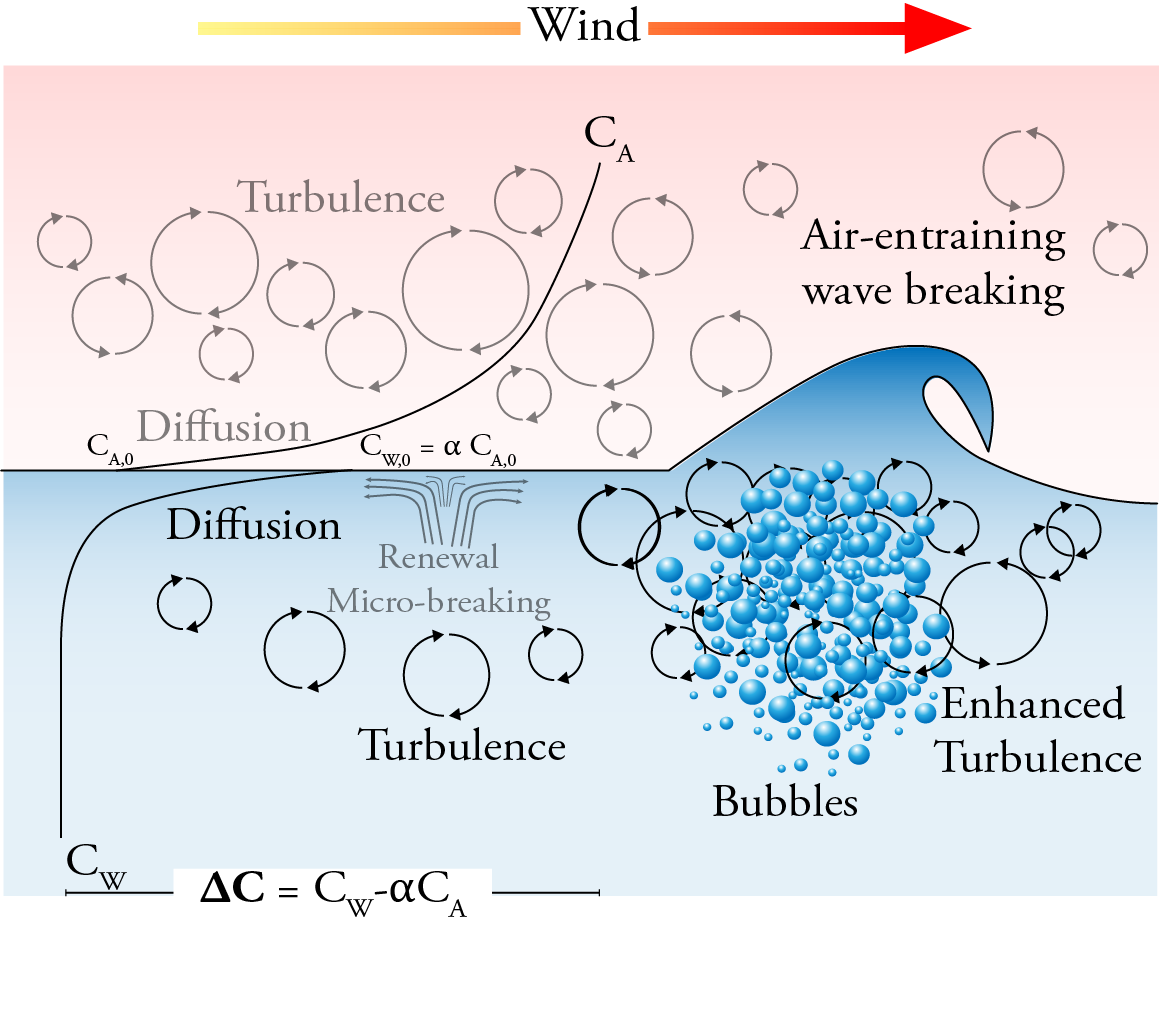Gas Transfer

The air-sea gas transfer can be divided into three processes: 1) a flux in the atmospheric viscous boundary layer between the bulk air and the air-side concentration boundary layer (CBL), 2) a flux across the interface between the air and water films, and 3) a flux in the water mass boundary layer between the aqueous CBL and bulk water. As the transfer across the interface occurs much faster than the transfer through either CBL, the air-sea flux is limited by the transport through the sublayers on either side of the interface. Whether the air or the water is most limiting depends on solubility. Sparingly soluble gases as CO$_2$ are water-side controlled.The bulk gas flux ($F_g$) across the air-sea interface can be expressed as the product of the gas transfer velocity ($k$) and the air-sea concentration difference ($\Delta C = C_w - \alpha C_a$, where $\alpha$ is the dimensionless Ostwald solubility coefficient): $F_g = k\Delta C = k K_0 \Delta p,$ where $K_0$ is the aqueous-phase solubility of the gas and $\Delta p$ the partial pressure difference. The gas transfer velocity both incorporates the diffusivity of the gas in water (which varies for different gases, temperatures and salinities) and represents the dependence of the flux on physical forcing mechanisms. \textit{k} is composed of both air and water phase transfer velocities and can be written in terms of water ($k_w^{-1}$) and air side ($k_a^{-1}$) resistance as: $k= \frac{1}{\frac{1}{k_w}+\frac{\alpha}{k_a}}.$